# Test: Limits And Continuity : Intuitive Approach - 3

## 40 Questions MCQ Test Quantitative Aptitude for CA CPT | Test: Limits And Continuity : Intuitive Approach - 3

Description
Attempt Test: Limits And Continuity : Intuitive Approach - 3 | 40 questions in 40 minutes | Mock test for CA Foundation preparation | Free important questions MCQ to study Quantitative Aptitude for CA CPT for CA Foundation Exam | Download free PDF with solutions
QUESTION: 1

Solution:
QUESTION: 2

Solution:
QUESTION: 3

### If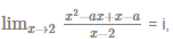= i, then which of the following is correct?

Solution:
QUESTION: 4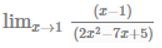is equal to

Solution:
QUESTION: 5

If f(x) = x for  0 ≤ x 1/2, f(x) = 1 for x = 1-x for 1/2<x<1 then at x =1/2 the function is

Solution:
QUESTION: 6

e-1/x[1+e1/x]-1 is

Solution:
QUESTION: 7

If f(x)=9x÷(x+2) for x<1, f(1)=3, f(x)=(x+3)x-1 for x>1, then in the interval (-3,3) the function is

Solution:
QUESTION: 8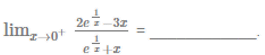Solution:
QUESTION: 9

Function f(x) = K.x-1  for x < 2
= x-k     for x ≥ 2
is continuous at x = 2
The value of 'k' is __________.

Solution:
QUESTION: 10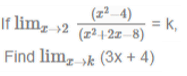Solution:
QUESTION: 11

Evaluate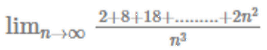Solution:
QUESTION: 12

Evaluate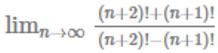Solution:
QUESTION: 13

The points of discontinuity of the function, F(x) =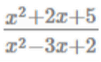are

Solution:
QUESTION: 14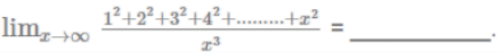Solution:
QUESTION: 15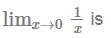Solution:
QUESTION: 16

Find limn→∞(2n-1)2(2n+1)-1 21-n

Solution:
QUESTION: 17

Find limn→∞[4n2 + 6n +2] ÷ 4n2

Solution:
QUESTION: 18

Find limn→∞[xn.(n+1)] ÷ [nx n+1 ]

Solution:
QUESTION: 19

Find limn→∞[n(n+2)] ÷ (n+1)2

Solution:
QUESTION: 20

Find limn→∞(n3 +a )[(n+1)3a]-1 (2n+1 +a ) (2n +a)-1

Solution:
QUESTION: 21

Find limn→∞(n2 +1)[(n+1)2 +1]-1 5n+1 5-n

Solution:
QUESTION: 22

Find limn→∞nn(n+1)-n-1 ÷ n-1

Solution:
QUESTION: 23

Find limn→∞2n-1 (10 +n) (9+n)-1 2-n

Solution:
QUESTION: 24

Find limn→∞(1+n-1)[1+2n)-1]-1

Solution:
QUESTION: 25

Find limn→∞[nn. (n+1)!] ÷ [n! (n+1)n+1]

Solution:
QUESTION: 26

Find limn→∞[(n+1)n+1. n-n-1 -(n+1).n-1]-n

Solution:
QUESTION: 27

3x2+2x-1 is continuous

Solution:
QUESTION: 28

Find limn→∞nn (1+n)-n

Solution:
QUESTION: 29

Find limn→∞[n!3n+1] ÷[3n(n+1)!]

Solution:
QUESTION: 30

The value of the limit when x tends to zero of the expression (1+n)1/n is

Solution:
QUESTION: 31

The value of the limit when x tends to zero of the expression (1+n)1/n is

Solution:
QUESTION: 32

The value of the limit when x tends to zero of the expression [(1+x)n-1] ]

Solution:
QUESTION: 33

The value of the limit when x tends to 2 of the expression (x-2)-1-(x2-3x+2)-1 is

Solution:
QUESTION: 34

The value of the limit when x tends to zero of the expression (ex-1)/x is

Solution:
QUESTION: 35

The value of the limit when n tends to infinity of the expression 2-n(n2+5n+6)[(n+4)(n+5)]-1 is

Solution:
QUESTION: 36

The value of the limit when x tends to zero of the expression (1+n)1/n is

Solution:
QUESTION: 37

Find  limn→∞[n1/2 + (n+1)1/2]-1 ÷ n-1/2

Solution:
QUESTION: 38

The value of the limit when x tends to zero of the expression [(a+x2)1/2

Solution:
QUESTION: 39

Find limn→∞ (2n-2)(2n+1)-1

Solution:
QUESTION: 40

Find limn→∞ [(n3 +1)1/2 - n 3/2] ÷ n3/2

Solution:Use Code STAYHOME200 and get INR 200 additional OFF Use Coupon Code